# RFEM – Online Manual

Online manuals, introductory examples, tutorials, and other documentation.

# 6.11 Free Variable Loads

General description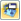With this function, you can define surface loads that act uniformly or variably over the depth and perimeter of surfaces. You can use free variable loads to, for example, model tank and wind loads for rotationally symmetric components.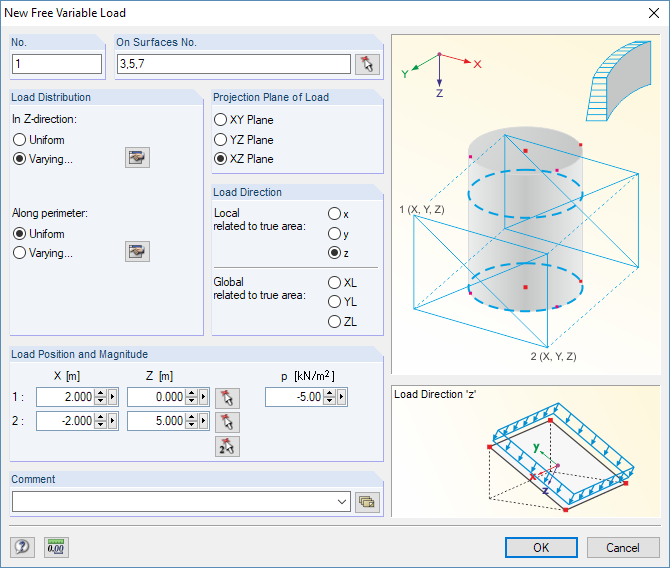Figure 6.45 New Free Variable Load dialog box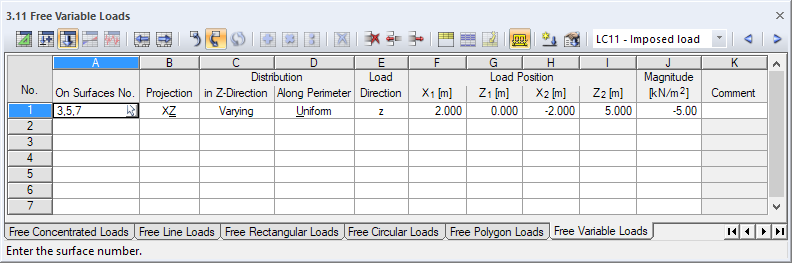Figure 6.46 Table 3.11 Free Variable Loads
On Surfaces No.

This text box manages the numbers of the surfaces on which the load acts. You can also select them graphically by using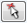.

Starting from the corner points defined by the Load Position, imaginary straight lines are "set" perpendicular to the projection plane. If these lines intersect any of the listed surfaces, the boundary line of the applied load is the connecting diagonal of both intersection points. In this way, it is possible to quickly allocate loads of a similar type to several surfaces.

Projection Plane

The load can be projected onto one of the global planes XY, YZ, or XZ. As described above, imaginary straight lines are generated, starting from the load positions and running perpendicular to the projection plane. The points of the rectangle are then assumed as a surface at all intersection points of the straight line.

The projection plane must not be perpendicular to a surface on which the load acts.

Load Distribution

The surface loads can act in Z-direction and/or Along perimeter as uniform or varying. You can define the parameters of varying loads in separate dialog boxes by using the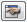buttons.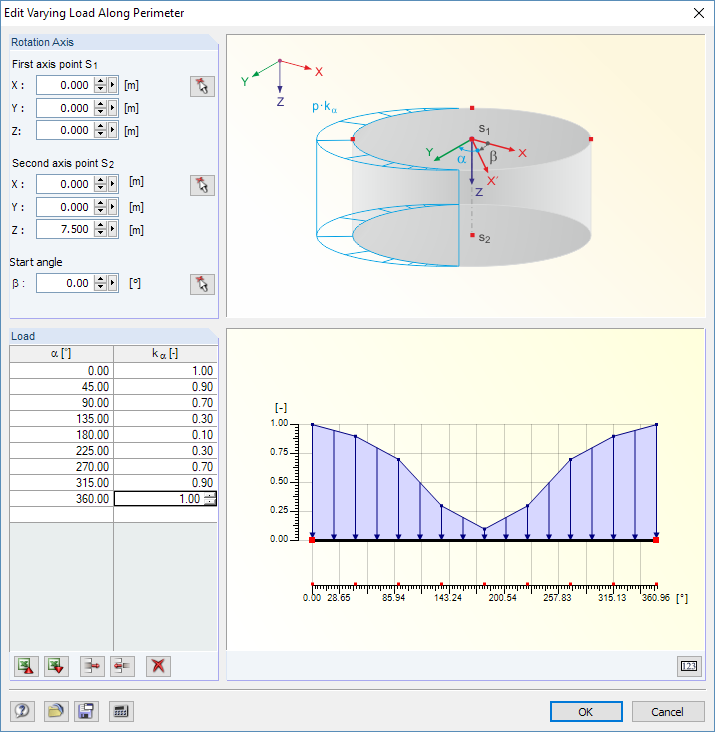Figure 6.47 Edit Varying Load Along Perimeter dialog box

The factor kα in Figure 6.47 establishes the relation to the load magnitude p of the initial dialog box (see below).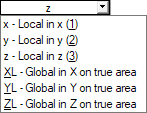Load Direction

The load can act in the direction of the local surface axes x, y, z or the global axes X, Y, Z. The load directions are described in Chapter 6.8.

Load Position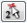The coordinates of a rectangle according to the dialog graphic determine in which area the load can act. You can define these corner points in the dialog box by using.

Magnitude

In this table column or text box, enter the numerical value of the area load. For a linearly varying distribution, you can define the load ordinates in the Edit Varying Load dialog box (see Figure 6.47).

You can find examples for free variable loads in the following articles of our Knowledge Base:
https://www.dlubal.com/en/support-and-learning/support/knowledge-base/000965
https://www.dlubal.com/en/support-and-learning/support/knowledge-base/000988